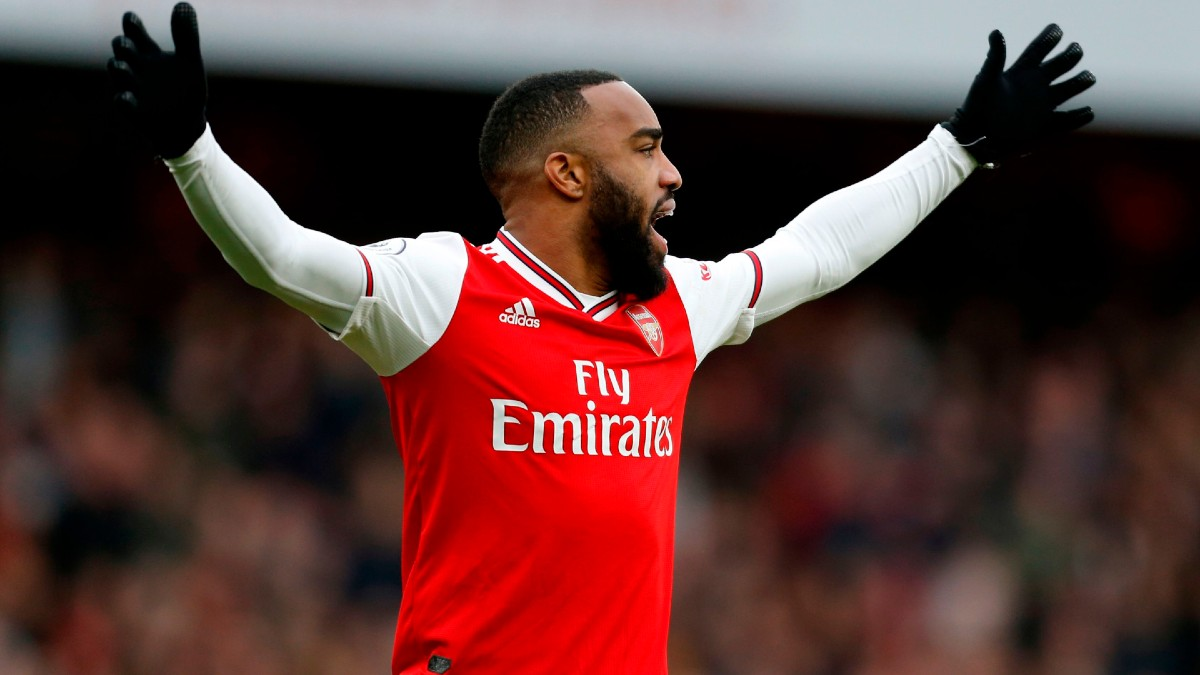# Decimal Odds in Sports Betting, ExplainedCredit:

IAN KINGTON/AFP via Getty Images. Pictured: Alexandre Lacazette

Decimal odds are the most popular type of odds around the world, and have two main advantages over American odds — they convert to probabilities much easier, and they’re slightly more intuitive once you’re familiar.

So how do decimal odds work?

Decimal odds represent the total return for every \$1 wagered, including the money you risked.

An American moneyline at -110 is 1.91 in decimal odds. Why?

Because for every \$1 you’re betting, you’re getting 91 cents back, plus the original dollar.

Therefore, any odds under 2.0 will represent a favorite. Any odds over 2.0 will be an underdog.

## Calculating Payouts with Decimal Odds

Calculating payouts with decimal odds is simple.

Just multiply your wager by the decimal odds, and you’re done.

Money Risked * Odds = Total Payout

Let’s say you want to bet \$10 on the Miami Dolphinsmoneyline (+160 in American odds) against the Buffalo Bills. In decimal odds, Miami will be 2.6.

\$10 x 2.6 = \$26

That \$26 represents the \$16 you’d profit from a Dolphins win, plus your original \$10 back.

Your pal Norman wants to bet \$10 on the Bills moneyline (-200 in American odds) in that same game. In decimal odds, that would be 1.5.

\$20 x 1.5 = \$30

If the Bills win, he’ll get \$20 back and profit \$10 for a total of \$30.

## Why Use Decimal Odds?

The biggest reason to switch to decimal odds is because the conversion to probabilities is so easy. It’s the same whether you’re working with an underdog or favorite.

Converting American odds to implied probabilities requires slightly different formulas for favorites and underdogs.

For decimals, it’s the same no matter what you’re betting.

Another reason to use decimal odds is that they’re based around betting \$1 and scale up and down easily, instead of being based on betting \$100 like American odds.

## How to Convert Decimal Odds to Implied Probability

I promised converting decimal odds to implied probabilities was easy. So here we go.

1 / Decimal Odds

That’s it!

What’s the implied probability of the Dolphins at +160 (or 2.5 in decimal odds)?

1 / 2.6 = 38.5%

The Bills at -200 (or 1.5 in decimal odds) would have an implied probability of 66.6%.

1 / 1.5 = 66.6%

## Converting American Odds to Decimal Odds

Decimal odds start to look familiar when you’ve seen them enough.

For example, an NFL point spread at -110 is 1.91. That will become second nature quickly.

But at first, you’ll need to do some conversion, especially for favorites. You can use our odds converter, or do it by hand.

Here’s the math:

For Positive Odds: 1 + (American odds / 100)

Let’s say you have American odds of +160 on the Dolphins. It’s easy enough.

1 + (160/100) = 2.6

For Negative Odds: 1 – (100 / American Odds)

So converting the Bills from -200 is slightly different.

1 – (100/-200) = 1.5

## Converting Decimal Odds to American Odds

If you still want to use American odds but only see a line in decimal odds at a European sportsbook, you can make the conversion.

To convert a decimal of 2.00 or higher: (Decimal odds – 1) * 100

In our Bills-Dolphins example, you’d plug 2.6 into the following formula to convert back to American odds.

(2.6 – 1) * 100 = +160

To convert a decimal of less than 2.00: (-100) / (Decimal Odds – 1)

For the Bills at 1.5, you’d do:

(-100) / (1.5 – 1) = -200

## Should I Switch to Decimal Odds?

U.S. sportsbooks will default to show American odds, but you can switch to show decimal odds instead. It’s usually in the top right corner once you’re logged in.

It’s a personal preference, but if you’re serious about using basic math like implied probabilities when you bet, decimal odds will save you the extra conversion steps.

## Start Betting Now

Ready to start betting? Head to DraftKings now for a \$1,000 sign-up bonus.